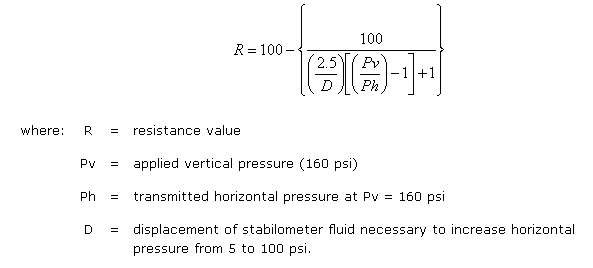# Resistance Value

The Resistance Value (R-value) test is a material stiffness test. The test procedure expresses a material’s resistance to deformation as a function of the ratio of transmitted lateral pressure to applied vertical pressure. It is essentially a modified triaxial compression test. Materials tested are assigned an R-value.

The R-value test was developed by F.N. Hveem and R.M. Carmany of the California Division of Highways and first reported in the late 1940’s. During this time rutting (or shoving) in the wheel tracks was a primary concern and the R-value test was developed as an improvement on the CBR test. Presently, the R-value is used mostly by State Highway Agencies (SHAs) on the west coast of the U.S.

The test procedure to determine R-value requires that the laboratory prepared samples are fabricated to a moisture and density condition representative of the worst possible in situ condition of a compacted subgrade. The R-value is calculated from the ratio of the applied vertical pressure to the developed lateral pressure and is essentially a measure of the material’s resistance to plastic flow. The testing apparatus used in the R-value test is called a stabilometer (identical to the one used in Hveem HMA mix design) and is represented schematically in Figure 1.

Values obtained from the stabilometer are inserted into the following equation to obtain an R-value:Some typical R-values are: